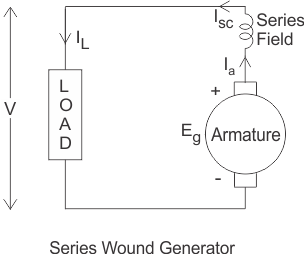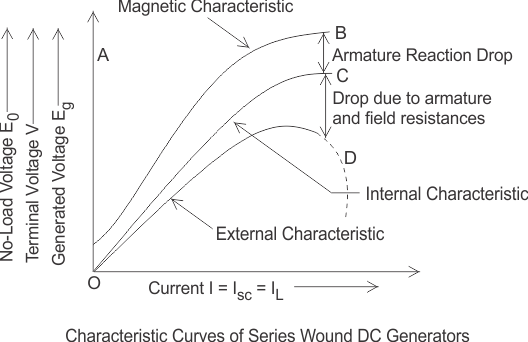# Characteristics of Series Wound DC Generator

In these types of generators the field windings, armature windings and external load circuit all are connected in series as shown in figure below.Therefore, the same current flows through armature winding, field winding and the load.
Let, I = Ia = Isc = IL
Here, Ia = armature current
Isc = series field current
There are generally three most important characteristics of series wound DC generator which show the relation between various quantities such as series field current or excitation current, generated voltage, terminal voltage and load current.

## Magnetic or Open Circuit Characteristic of Series Wound DC Generator

The curve which shows the relation between no load voltage and the field excitation current is called magnetic or open circuit characteristic curve. As during no load, the load terminals are open circuited, there will be no field current in the field since, the armature, field and load are series connected and these three make a closed loop of circuit. So, this curve can be obtained practically be separating the field winding and exciting the DC generator by an external source.

Here in the diagram below AB curve is showing the magnetic characteristic of series wound DC generator. The linearity of the curve will continue till the saturation of the poles. After that there will be no further significant change of terminal voltage of DC generator for increasing field current. Due to residual magnetism there will be a small initial voltage across the armature that is why the curve started from a point A which is a little way up to the origin O.

## Internal Characteristic of Series Wound DC Generator

The internal characteristic curve gives the relation between voltage generated in the armature and the load current. This curve is obtained by subtracting the drop due to the demagnetizing effect of armature reaction from the no load voltage. So, the actual generated voltage (Eg) will be less than the no load voltage (E0). That is why the curve is slightly dropping from the open circuit characteristic curve. Here in the diagram below OC curve is showing the internal characteristic or total characteristic of the series wound DC generator.

## External Characteristic of Series Wound DC Generator

The external characteristic curve shows the variation of terminal voltage (V) with the load current (IL). Terminal voltage of this type of generator is obtained by subtracting the ohomic drop due to armature resistance (Ra) and series field resistance (Rsc) from the actually generated voltage (Eg).
Terminal voltage V = Eg – I(Ra + Rsc)
The external characteristic curve lies below the internal characteristic curve because the value of terminal voltage is less than the generated voltage. Here in the figure OD curve is showing the external characteristic of the series wound DC generator.